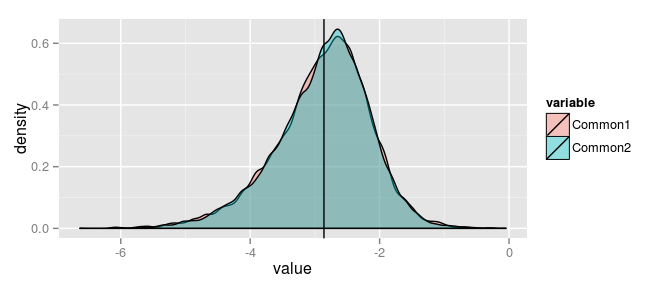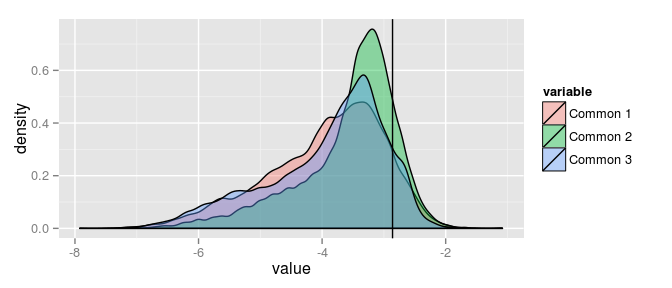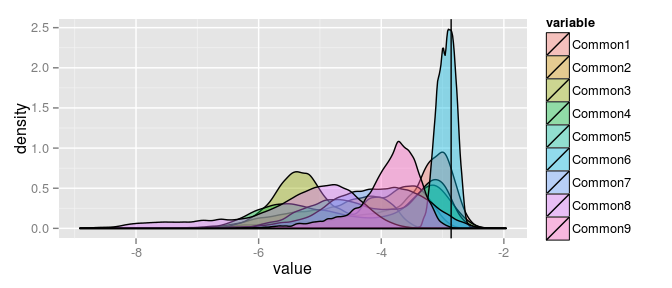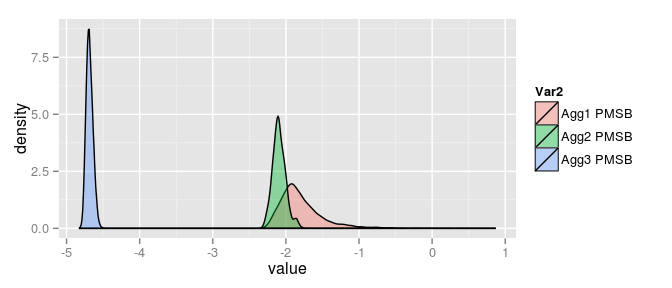#Updates and Research from Steve Bronder

The purpose of this site is to give current information on Steve Bronder's (me) research and personal life

Panel Analysis of Nonstationarity in Idiosyncratic and Common Components

The purpose of this package is to perform the Panel Analysis of Nonstationarity in Idiosyncratic and Common Components from Bai and Ng (2004,2010). When working with large dimensional panels, standard pooled and aggregated nonstationarity tests tend to over-reject null hypothesis due to:

1. Curse of dimensionality
2. Cross-Correlation in panel structure
3. Weak strength to Large N or large T

Instead of testing the data directly, PANIC performs a factor model to derive the common and idiosyncratic components of the panel. By using the BIC3 from Bai and Ng (2004) it is possible to determine the number of common components in panels that reduce cross correlation in the error term. In this vignette we will perform PANIC on three aggregate levels of National Income and Product Accounts in order to test these aggregates for aggregation bias

## Vignette Info

This vignette will use the functions panic10() and panic04() availabe through the PANICr package. This package can be downloaded from github or from CRAN by using the install_github() function available in the package devtools.

library(devtools)
install_github("stevo15025/PANICr")
install.packages("PANICr")

These functions perform a factor model on each level of aggregation, derive the common and idiosyncratic components, and then perform several pooled test statistics. One of several benefits of PANIC is that by reducing cross-correlation we allow valid pooling of individual statistics and so panel tests can be run that have reasonable strength.

Performing the factor analysis using BIC3, the criteria for determining the number of factors in our approximate factor model, allows us to determine whether the nonstationarity is pervasive, variable specific, or both.

## Data

The data we use is gathered from the Price Indexes for Personal Consumption Expenditures by Type of Product available from the BEA. The data is monthly from 1959 to 20131. At this point we run the data through X-132.

After extracting each sector we divide them up into three seperate levels of aggregation from highest level of aggregation to lowest. To turn this dataset into year on year inflation we perform $$log(p_{t}/p_{t-12})$$. The data is available already cleaned and manipulated as NIPAagg1, NIPAagg2, and NIPAagg3, respectively. The dimensions of the aggregates are (N=12,T=639), (N=46,T=639), (N=160,T=639), respectively.

## Model

Consider a factor analytic model:

$$X_{it} = D_{it} + \lambda_{i}' F_{t} + e_{it}$$

Where $$D_{it}$$ is a polynomial trend function, $$F_{t}$$ is an $$r\times{1}$$ vector of common factors, and $$\lambda'_{i}$$ is a vector of factor loadings. The panel $$X_{it}$$ is the sum of a deterministic component $$D_{it}$$ , a common component $$\lambda_{i}' F_{t}$$, and an error $$e_{it}$$ that is largely idiosyncratic. A factor model with $$N$$ variables has $$N$$ idiosyncratic components, but a smaller number of common factors.

$$D_{it}$$ can be modeled by $$P$$. In PANIC 2004, When the number of common factors is greater than one, $$P=1$$ and the deterministic trend has an individual specific fixed effect and time trend. When the number of common factors is equal to one, $$P=0$$ is an individual specific fixed effect. When the number of common factors is zero, $$P=0$$ is neither.

PANIC 2010 examines the data with ADF models A, B, and C. A assumes no deterministic component, B assumes a constant to allow for a fixed effect, and C allows a constant and a trend. Note that this is different than P as P is a data generating process while Models A, B, and C impose these constraints inside of the ADF test.

The benefit of this factor model is that, if the number of factors has been correctly determined, the error term will be largely idosyncratic and the common components will explain the largest variance of the data. To determine the approximate number of factors we use the BIC3 from Bai and Ng (2002) such that:

$$BIC3 = V(k,\hat{F}^k)+k\hat{\sigma}^2 + \frac{(N+T-k)ln(NT)}{NT}$$

$$(k,\hat{F}^k)$$ is the average residual variance when k factors are assumed for each cross-section unit. $$\hat{\sigma}^2$$ is the mean of the error term squared over N and T.

Once we have this model we perform ADF style pooled tests on the idiosyncratic and common components. panic04 and panic10 ask for nfac, the number of estimated factors, k1, the maximum lag allowed in the ADF test, and jj, the criteria to determine the number of factors in our approximate factor model. To determine the lag of the ADF test Bai and Ng (2002) suggest $$4(\sqrt{\frac{T}{100}})$$.

I utilize Bai and Ng’s (2002) third information criterion to determine the number of common factors. nfac is weak to underestimation so it is suggested to overestimate the number of factors. jj is an Integer 1 through 8. Choices 1 through 7 are respectively, IC(1), IC(2), IC(3), AIC(1), BIC(1), AIC(3), and BIC(3), respectively. Choosing 8 makes the number of factors equal to the number of columns whose sum of eigenvalues is less than or equal to .5. panic10() also has the option to run models on demeaned or non-demeaned data (TRUE or FALSE) which will return models A and B in the first case and C in the second.

With this information it is now appropriate to start running our tests.

  library(PANICr)
library(MCMCpack)
library(Revobase)
data(NIPAagg1)
data(NIPAagg2)
data(NIPAagg3)

agg1.04 <- panic04(NIPAagg1,9,7,8)

### Aggregate One Results from PANIC 2004

Test Values
Pooled Demeaned 151.287625586434 18.3723862242089
Pooled Idiosyncratic 131.157425663919 15.4668421381828
Pooled Cointegration test 197.117010015667 24.9872880834621

## Common Test

-5.719374 -4.345388

Above is what all of the results will look like for panic04(). These tests are based on ADF tests and so our null hypothesis is non-stationarity and our alternative is stationarity. Pooled Demeaned is our pooled test statistic on the demeaned dataset. With a critical value of 2.87 we reject this test statistic and conclude stationarity.

Our pooled idiosyncratic test is the pooled test on the idiosyncratic component from our factor analysis. This test has a critical value of 1.64 (Bai and Ng(2004)) at the .05 percent level and so we reject our null and conclude stationarity. For the Pooled Cointegration test we assume a null that the estimated number of common factors is correct and an alternative of the number of common factors chosen is not equal to the true number of common factors.

If we have properly estimated the number of common factors then there should be no cointegration inside of our common components. Cointegration is a problem because it puts our ADF tests on the common components into question. For this test statistic we refer back to the table on page 1135 of Bai and Ng (2004). For convenince, I have posted these critical values below.

## Critical Values for Cointegration test

m .01 .05 .10
1 -20.151 -13.730 -11.022
2 -31.621 -23.535 -19.923
3 -41.064 -32.296 -28.399
4 -48.501 -40.442 -36.592
5 -58.383 -48.617 -44.111
6 -66.978 -57.040 -52.312

And so for our above results we look at m = 2, because we have two common components. We reject the null at the .05 percent level, but do not reject at the .01 percent level. Our test on the common components has a critical value of -2.86 at the .05 percent level. For our example above we reject the null and assume stationarity. Below we repeat this process for aggregates two and three.

agg2.04 <- panic04(NIPAagg2,9,7,8)

### Aggregate Two Results from PANIC 2004

Test Values
Pooled Demeaned 611.71868977349 38.8866151821883
Pooled Idiosyncratic 522.753968510507 32.2555763707432
Pooled Cointegration test 490.814016498057 29.8749129074792

## Common Test

-6.281520 -5.915479 -4.683622

agg3.04 <- panic04(NIPAagg3,5,7,3)

### Aggregate Three Results from PANIC 2004

Test Values
Pooled Demeaned 2115.1699605466 73.452641227304
Pooled Idiosyncratic 1757.4869117784 58.9466781059138
Pooled Cointegration test 2799.94347308076 101.223874316303

## Common Test

-5.935561 -6.873747 -7.459283 -7.441815 -6.793798

In 2010 Bai and Ng released two new tests. One test estimates the pooled autoregressive coefficient, and one uses a sample moment in order to account for structural breaks. The estimate of the pooled autoregressive coefficient is based on Moon and Perron and from here on called MP. This test has three different models dubbed A, B, and C.

Model C assumes an incidental trend model as well as a fixed effect, Model B imposes a fixed effect, and Model A assumes no incendental trend or fixed effect. These tests are are asymptotically normal and reject our null hypothesis of nonstatinarity at postive or negative 1.96.

The function panic10() also includes a pooled autoregressive test that is based on a bias corrected pooled autoregressive coefficient for the idiosyncratic errors estimated by PANIC. This test is asymptotically normal and based on a demeaned data generating process (DGP).

The test that accounts for structural breaks is known as the PMSB test as it is a panel version of the Sargan-Bhargava test (MSB). An interesting thing to note about this test is the the critival value is degenerative. This means that instead of rejecting the null of stationarity after we go past a critical value we reject the null if we go below a critical value.

The function panic10() contains a parameter demean. When this is set to true the function using a data generating process (DGP) that demeans the data and only runs model C of the MP tests, the pooled tests, PMSB, and the LM test from original PANIC (2004).

Setting this parameter to false runs a DGP that does not demean the data and returns model A and B of the MP test, PMSB, and the pooled ADF test of PANIC 2004. For each test you receive two test statistics. As common with tests of nonstationarity, we must reject both test statistics in order to conclude stationarity.

agg1.10.d <- panic10(NIPAagg1,12,7,7,TRUE)

### Aggregate One Results from PANIC 2010 Demeaned Tests

Pool Test P MP Test Model C
Pa 1.50961575098886 ta -3.79874618927826
Pb 1.78373520173856 tb -2.59128210550658
PMSB rho1 04 Pool LM
1.211956 0.9957968 -0.3808114

As an example, for the demeaned tests above, we do not reject the null for the PMSB test, but we do reject for MP model C test and the pooled test. However, for PANIC 2004 we do not reject the null. This is most likely due to the fact the the LM does not take into account the structural shift in the data. Below are the results for aggregates two and three as well as the asymptotic critical percentiles for the PMSB. I was unable to find the critical values for Bai and Ng’s 2010 test, so we use the critical values from Stock (1990) for the MSB test.

#### Asymptotic critical percentiles for the PMSB

Percentile Demeaned Detrended
.025 .17405 .015250
.05 .19144 .16449
.10 .21426 .18050
agg2.10.d <- panic10(NIPAagg2,12,7,7,TRUE)

### Aggregate Two Results from PANIC 2010 Demeaned Tests

Pool Test P MP Test Model C
Pa -25.8547458418636 ta -2.26642977540598
Pb -10.3350902614155 tb -1.10444796408497
PMSB rho1 04 Pool LM
-4.069127 0.9984711 27.98281
agg3.10.d <- panic10(NIPAagg3,12,7,8,TRUE)

### Aggregate Three Results from PANIC 2010 Demeaned Tests

Pool Test P MP Test Model C
Pa -36.7995444340426 ta -3.44442550007929
Pb -15.017863712126 tb -1.58906392647549
PMSB rho1 04 Pool LM
-6.039356 0.998452 52.06343
agg1.10.nd <- panic10(NIPAagg1,12,7,8,FALSE)

### Aggregate One Results from PANIC 2010 Non-Demeaned Tests

MP Model A Model B
ta -18.6986081207263 0.496952725573856
tb -5.83235690939428 0.242043809710246
PMSB rho1 04 Pool ADF
-2.088406 1.000497 14.97401

Above are the results of PANIC 2010’s non-demeaned DGP for aggregate one. We reject for the PMSB test and MP test Model A, and pooled ADF test for PANIC 2004. However, we do not reject for model B.

agg2.10.nd <- panic10(NIPAagg2,12,7,8,FALSE)

### Aggregate Two Results from PANIC 2010 Non-Demeaned Tests

MP Model A Model B
ta -41.6496402167829 -0.687013376283988
tb -11.6726242443272 -0.243108562817226
PMSB rho1 04 Pool ADF
-3.752921 0.9996068 30.84134
agg3.10.nd <- panic10(NIPAagg3,12,7,8,FALSE)

### Aggregate Three Results from PANIC 2010 Non-Demeaned Tests

MP Model A Model B
ta -55.4162521481366 -2.29876504766966
tb -15.7271993582764 -0.714690391494632
PMSB rho1 04 Pool ADF
-5.146306 0.9990782 57.71948

## Interpreting Results

What does this mean? All of these tests are on the idiosyncratic component so can only tell us whether or not nonstationarity lies outside of the common component. It is important to run panic04() in order to test whether or not the common compoenents are nonstationary.

## MCMC functions for PANIC 2004

One thing we may be curious about is the distribution of each of our test statistics. For example, since we are studying aggregation bias, if the probability of rejecting the null aggregate one is much higher than for aggregate two or three this may be a good indicator as to whether aggregation bias exists within the data. To find these distributions we perform an MCMC technique known as gibbs sampling.

Gibbs sampling is a Markov chain Monte Carlo algorithm for obtaining a sequence of observations which are approximated, for our case, from a inverse gamma distribution. We use this technique to derive the marginal distribution for each test statistic as a means of statistical inference.

To ensure stationarity of our markov chain and independence of resamples during our Gibbs process, we burn 50000 chains before starting the process of gathering samples (mcmc=100000). In addition, we thin the samples by only taking every tenth sample. In the below code, seed specifies where we start in R’s random number generator. lambda.start is the starting values for the factor loading matrix lambda. psi.start is the starting values for the uniqueness. l0 is the means of the independent normal prior on the factor loadings. L0 is the precisions (inverse variances) of the independent normal prior on the factor loadings. For the rest of the these paramater specifications please see the help file for MCMCpack’s MCMCfactanal() function.

mcmcagg1.04 <- MCMCpanic04(NIPAagg1, 9, 7, 8, burn = 50000, mcmc = 100000, thin = 10,
verbose = 0,seed = NA, lambda.start = NA, psi.start = NA,
l0 = 0, L0 = 0, a0 = 0.001, b0 = 0.001, std.var = TRUE)
mcmcagg2.04 <- MCMCpanic04(NIPAagg2, 9, 7, 8, burn = 50000, mcmc = 100000, thin = 10,
verbose = 0,seed = NA, lambda.start = NA, psi.start = NA,
l0 = 0, L0 = 0, a0 = 0.001, b0 = 0.001, std.var = TRUE)

With large datasets caution should be used on the number of variables allowed in the factor model. A large number can cause the function to run extremely slow and be memory intensive. We thin twice as much for our third aggregate in order to avoid errors. Currently (October 19th, 2014) my laptops 8GB’s of RAM is not sufficient to perform the tests on the third aggregate. We can see what the function would look like here.

mcmcagg3.04 <- MCMCpanic04(NIPAagg3, 9, 7, 8, burn = 80000, mcmc = 100000, thin = 10,
verbose = 0,seed = NA, lambda.start = NA, psi.start = NA,
l0 = 0, L0 = 0, a0 = 0.001, b0 = 0.001, std.var = TRUE)

After running aggregates one and two we receive the test statistics for each chain of the MCMC. turning these back into mcmc objects allows us to use the coda packages built in functions for analyzing markov chains. We use thesummary() function from the coda package for MCMCs. The second list of this function gives us the quantiles for each chain.

adf.mcmc1 <- as.mcmc(mcmcagg1.04$adf.mcmc) adf.mcmc2 <- as.mcmc(mcmcagg2.04$adf.mcmc)
adf.mcmc3 <- as.mcmc(mcmcagg3.04$adf.mcmc) summary(adf.mcmc1)[] ## 2.5% 25% 50% 75% 97.5% ## adf50a 125.231057 125.231057 125.231057 125.231057 125.231057 ## adf50b 14.611445 14.611445 14.611445 14.611445 14.611445 ## adf30a 40.492286 73.013338 93.852007 114.141295 146.480662 ## adf30b 2.380457 7.074466 10.082269 13.010775 17.678561 ## Common1 -4.555162 -3.297058 -2.802654 -2.388478 -1.596328 ## Common2 -4.503032 -3.297056 -2.797343 -2.385216 -1.601497 summary(adf.mcmc2)[] ## 2.5% 25% 50% 75% 97.5% ## adf50a 601.744908 601.744908 601.744908 601.744908 601.744908 ## adf50b 38.143213 38.143213 38.143213 38.143213 38.143213 ## adf30a 424.355445 488.002515 515.543446 541.600056 581.584314 ## adf30b 24.921383 29.665356 31.718136 33.660281 36.640531 ## Common1 -6.084734 -4.534410 -3.790174 -3.246464 -2.479543 ## Common2 -5.668480 -3.916884 -3.373164 -3.032195 -2.436369 ## Common3 -6.162122 -4.490731 -3.682528 -3.228555 -2.566131 summary(adf.mcmc3)[] ## 2.5% 25% 50% 75% 97.5% ## adf50a 2799.943473 2799.943473 2799.943473 2799.943473 2799.943473 ## adf50b 101.223874 101.223874 101.223874 101.223874 101.223874 ## adf30a 1572.650136 1736.854054 1804.574554 1865.078377 1949.217731 ## adf30b 51.450557 58.109905 60.856334 63.310088 66.722390 ## Common1 -6.157066 -3.948064 -3.285173 -2.999441 -2.666419 ## Common2 -6.210889 -4.919153 -4.161510 -3.587016 -3.070640 ## Common3 -6.152827 -5.508712 -5.152452 -4.372105 -3.699252 ## Common4 -6.338197 -5.430761 -4.207801 -3.236666 -2.714977 ## Common5 -5.739598 -4.786943 -3.954987 -3.209999 -2.773362 ## Common6 -3.227658 -3.046328 -2.930740 -2.829335 -2.681425 ## Common7 -5.733256 -4.585485 -4.049665 -3.542639 -2.817319 ## Common8 -7.924401 -5.803500 -5.123694 -4.659119 -3.977446 ## Common9 -5.289584 -4.093804 -3.771082 -3.530861 -3.152275 Investigating the quantiles for each test statistic gives us the critical value at each. for adf50a and b the test statistic holds as in bai and ng (2004) and the value is the same for each quantile. For ADF30a and b we see the quantiles for each distribution are very different. Similarly, for each of the common components aggregate one appears to have a much higher chance of rejecting the test statistic. library(ggplot2) library(reshape2) melt.adf1.mcmc <- melt(mcmcagg1.04$adf.mcmc[,5:6])
## No id variables; using all as measure variables
adf.density1 <- ggplot(data = melt.adf1.mcmc, aes(x=value)) + geom_density(aes(fill=variable), alpha = 0.4)+geom_vline(xintercept=-2.86)

adf.density1Above and below are graphical representations of the probability distributions of our common tests for aggregate 1 and 2, respectively. This is a nice feature of MCMC functions as we can graphically display what the probability of rejecting our critical value (black line) for each component of each aggregate. It’s pretty clear that aggregate 1 is much less likely to reject our null hypotheis, while aggregate two is much more likely.

## No id variables; using all as measure variables## No id variables; using all as measure variablesNow we run the MCMC version of PANIC10’s demeaned test statistics. Due to computational constraints we cannot currently run aggregate 3.

mcmcagg1.10nd<- MCMCpanic10(NIPAagg1, 9, 7, 8, burn = 50000, mcmc = 100000, thin = 10,
verbose = 0,seed = NA, lambda.start = NA, psi.start = NA,
l0 = 0, L0 = 0, a0 = 0.001, b0 = 0.001, std.var = TRUE,demean=FALSE)
mcmcagg2.10nd<- MCMCpanic10(NIPAagg2, 9, 7, 8, burn = 50000, mcmc = 100000, thin = 10,
verbose = 0,seed = NA, lambda.start = NA, psi.start = NA,
l0 = 0, L0 = 0, a0 = 0.001, b0 = 0.001, std.var = TRUE,demean=FALSE)
mcmcagg3.10nd<- MCMCpanic10(NIPAagg3, 9, 7, 8, burn = 80000, mcmc = 90000, thin = 10,
verbose = 0,seed = NA, lambda.start = NA, psi.start = NA,
l0 = 0, L0 = 0, a0 = 0.001, b0 = 0.001, std.var = TRUE,demean=FALSE)

Similar to PANIC04’s MCMC function we can turn our results into mcmc objects and run the summary function from CODA to get the quantiles for each.

adf.mcmc1.10nd <- as.mcmc(mcmcagg1.10nd)
adf.mcmc2.10nd <- as.mcmc(mcmcagg2.10nd)
adf.mcmc3.10nd <- as.mcmc(mcmcagg3.10nd)

summary(adf.mcmc1.10nd)[]
##                    2.5%          25%          50%          75%
## model A ta  -25.6086277  -15.4764825  -10.4205212   -6.3612674
## model A tb   -6.8382881   -5.1563662   -4.1272577   -3.0914823
## model B ta -693.1938957 -487.0976912 -356.2937193 -235.6158189
## model B tb  -23.1988273  -18.2119190  -15.8554987  -13.5050017
## PMSB         -2.1865478   -1.9880077   -1.8584603   -1.6737414
## rho           0.2986072    0.4648425    0.5880048    0.7225548
##                   97.5%
## model A ta   -1.6982237
## model A tb   -1.2256493
## model B ta -100.5458183
## model B tb   -8.9986663
## PMSB         -0.9919983
## rho           0.8773361
summary(adf.mcmc2.10nd)[]
##                    2.5%          25%          50%          75%
## model A ta  -42.4917344  -37.0618129  -33.2659012  -29.6033438
## model A tb   -8.8366926   -8.1918255   -7.7346271   -7.2604858
## model B ta -769.2484042 -577.6175709 -492.9194886 -419.5287162
## model B tb  -37.6510205  -31.3922793  -28.2508684  -24.8387235
## PMSB         -2.2557954   -2.1515108   -2.0983560   -2.0367805
## rho           0.4969162    0.5987158    0.6430157    0.6815366
##                   97.5%
## model A ta  -21.5014310
## model A tb   -6.0665416
## model B ta -296.5864155
## model B tb  -17.5478986
## PMSB         -1.8725339
## rho           0.7323525
summary(adf.mcmc3.10nd)[]
##                     2.5%           25%           50%           75%
## model A ta   -55.5288911   -53.4504601   -51.8555879   -49.7183988
## model A tb   -15.1184973   -14.7884171   -14.5579304   -14.2407958
## model B ta -1634.4972642 -1475.8058616 -1403.9599757 -1340.3346551
## model B tb   -75.1448623   -70.0289513   -66.8493457   -63.2883724
## PMSB          -4.7614072    -4.7143087    -4.6860978    -4.6524817
## rho            0.4440633     0.4777469     0.4922769     0.5047845
##                   97.5%
## model A ta   -43.871506
## model A tb   -13.346731
## model B ta -1211.894313
## model B tb   -55.133808
## PMSB          -4.584474
## rho            0.526839

Below are the probability distributions for the PMSB test.

PMSB.test <- cbind(mcmcagg1.10nd[,5],mcmcagg2.10nd[,5],mcmcagg3.10nd[,5])
## Warning in cbind(mcmcagg1.10nd[, 5], mcmcagg2.10nd[, 5], mcmcagg3.10nd[, :
## number of rows of result is not a multiple of vector length (arg 3)
colnames(PMSB.test) <- c("Agg1 PMSB","Agg2 PMSB","Agg3 PMSB")

melt.adf1.mcmc10 <- melt(PMSB.test)

adf.density1.10 <- ggplot(data =melt.adf1.mcmc10, aes(x=value)) + geom_density(aes(fill=Var2),alpha = 0.4)

adf.density1.10`1. T = 660

2. X-13 is a software program available from the U.S. Census Bureau that seasonally adjusts multiple time series using X-13ARIMA-SEATS process